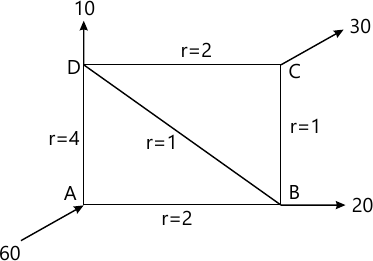MORE IN Fluid Mechanics - 2
MU Civil Engineering (Semester 4)
Fluid Mechanics - 2
May 2014
Total marks: --
Total time: --
INSTRUCTIONS
(1) Assume appropriate data and state your reasons
(2) Marks are given to the right of every question
(3) Draw neat diagrams wherever necessary

Solve any four
1 (a) Define mach number. What is the significance in compressible fluid flow.
5 M
1 (b) Derive Dupit's equation.
5 M
1 (c) Explain water hammer with control measure.
5 M
1 (d) Explain prandtl's mixing length theory.
5 M
1 (e) What is kinetic energy correction factor and momentum correction factor.
5 M

2 (a) Three pipes of diameters 300mm, 200 mm, and 400 mm and length 450 m,255 m and 315 m respectively are connected in series. The difference in water surface levels in two tanks is 18m. Determine the rate of flow of water if co-efficients of friction are 0.0075, 0.0078 and 0.0072 respectively considering.
i) Minor losses also and
ii) Neglecting minor losses.
10 M
2 (b) A 300 mm diameter horizontal pipe is suddenly enlarged to 600mm. The rate of flow of water through pipe is 0.5m3/s. If the intensity of pressure in smaller pipe is 120 KN/m2 determine.
i) Loss if head due to sudden enlargement.
ii) Intensity of pressure in large pipe.
iii) Power lost due to enlargement.
10 M

3 (a) Calculate dicharge in each pipe of network as shown in fig. The pipe network consists of 5 pipes. The head loss 'hf' in pipe is given by hf=rQ2. The ralues of 'r' for various pipe and also inflow or outflows at node are shown in figures.10 M
3 (b) A nozzle fitted to a pipe 100mm diameter and 250m long with coefficient of friction as 0.01. If head available at nozzle is 120. find maximum power transmitted by jet of water freely out of a nozzle and diameter of the nozzle.
10 M

4 (a) Prove relationship for one dimensioal compressible flow.
$\dfrac{dA}{A}=\dfrac{dP}{PV^{2}}\left [ 1-M^{2} \right ]$
10 M
4 (b) A supersonic aircraft files at an altitude of 3 km where temperature is 4°C. Determine the speed of aircraft if its sound is heared 5 second after its passage over the head of an observer.
Take R=287 J/kg0k and k=1.4.
10 M

5 (a) Derive an expression for the coefficient of viscosity in case of dashpot arrangement.
10 M
5 (b) A lubricating oil of viscosity 1 poise and specific gravity 0.9 is pumped through a 30mm diameter pipe. If the pressure drop per meter length of pipe is 20 KN/m2 determine.
i) Mass flow rate in kg/min
ii) The shear number of flow and check the flow
iii) Reynolds number of flow and check the flow.
iv) The power required per 50m length of the pipe to maintain the flow.
10 M

6 (a) Describe in detail hydro-dynamically smooth and rough boundaries. What is karman- Prandtl equation for hydrodynamical rough boundaries.
8 M
6 (b) Explain Moody's diagram.
5 M
6 (c) A pipe of diameter 100 mm, carrying water, the velocities at pipe centre and 35 mm from pipe centre are found tube 2.7m/s and 2.4m/s respectively. Find the wall shearing stress.
7 M

More question papers from Fluid Mechanics - 2